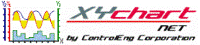XY Chart NET 3 Control Reference
SetDeltaCrosshairsPosition Method

Use this method to set the delta crosshairs to a specific x/y location on the chart.   This method is only available in the delta crosshairs mode (see SetDeltaCrosshairsMode).

Syntax
 [Visual Basic] Sub SetDeltaCrosshairsPosition(XCrossCoordinate As Double,                                                  YCrossCoordinate As Double,                                                  XScaleIndex As Integer,                                                  YScaleIndex As Integer,                                                  XDeltaAs Double,                                                  YDelta As Double) Sub SetDeltaCrosshairsPosition(X1CrossCoordinate As Double,                                                  Y1CrossCoordinate As Double,                                                  X1ScaleIndex As Integer,                                                  Y1ScaleIndex As Integer,                                                  X2CrossCoordinate As Double,                                                  Y2CrossCoordinate As Double,                                                  X2ScaleIndex As Integer,                                                  Y2ScaleIndex As Integer) [C#] void XYChartNETCtl.SetDeltaCrosshairsPosition(double XCrossCoordinate,                                                                            double YCrossCoordinate,                                                                            int XScaleIndex,                                                                            int YScaleIndex,                                                                            double XDelta,                                                                            double YDelta) void XYChartNETCtl.SetDeltaCrosshairsPosition(double X1CrossCoordinate,                                                                            double Y1CrossCoordinate,                                                                            int X1ScaleIndex,                                                                            int Y1ScaleIndex,                                                                            double X2CrossCoordinate,                                                                            double Y2CrossCoordinate,                                                                            int X2ScaleIndex,                                                                            int Y2ScaleIndex) [C++] void SetDeltaCrosshairsPosition(double, double, int, int, double, double) void SetDeltaCrosshairsPosition(double, double, int, int, double, double, int, int)

Parameters
XCrossCoordinate, X1CrossCoordinate
Indicates the plot's x-value to set the first vertical crosshair at.
X2CrossCoordinate
Indicates the plot's x-value to set the second vertical crosshair at.
YCrossCoordinate, Y1CrossCoordinate
Indicates the plot's y-value to set the horizontal crosshair at.
Y2CrossCoordinate
Indicates the plot's y-value to set the second horizontal crosshair at.
XScaleIndex, X1ScaleIndex
Indicates which x-axis the XCrossCoordinate (or X1CrossCoordinate) value pertains to.   Valid range: 0 to NumXScales - 1.
X2ScaleIndex
Indicates which x-axis the X2CrossCoordinate value pertains to.   Valid range: 0 to NumXScales - 1.
YScaleIndex, Y1ScaleIndex
Indicates which y-axis the YCrossCoordinate (or Y1CrossCoordinate) value pertains to.   Valid range: 0 to NumYScales - 1.
Y2ScaleIndex
Indicates which y-axis the Y2CrossCoordinate value pertains to.   Valid range: 0 to NumYScales - 1.
XDelta
Indicates the delta between the first and second vertical crosshairs.
YDelta
Indicates the delta between the first and second horizontal crosshairs.
Remarks
The delta crosshairs characteristics are determined by the Delta Crosshairs property.
Example
```' XYChartNETCtl1 is the name of the XY Chart NET control instance placed on the form.

Dim xCrossCoord As Double
Dim yCrossCoord As Double
Dim xScaleIdx As Integer
Dim yScaleIdx As Integer

xScaleIdx = 0      'X-axis scale index the crosshair's x-coord will be set against
yScaleIdx = 0      'Y-axis scale index the crosshair's y-coord will be set against
xCrossCoord = 24   'Vertical crosshair position
yCrossCoord = 500  'Horizontal crosshair position

'Position crosshairs; a Refresh call is not required
XYChartNETCtl1.SetDeltaCrosshairsMode(True)
XYChartNETCtl1.SetDeltaCrosshairsPosition(xCrossCoord, yCrossCoord, xScaleIdx, yScaleIdx, 10, 100)
```
```
```
```
```